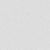Submit your work, meet writers and drop the ads. Become a member
Jul 2018
For translational
invariant functions
The Lebesgue measure is an            example of such a function;
­   In geometry, a translation "slides"

a thing by a: Ta(p) = p + a.

In physics and mathematics,
continuous translational symmetry
is the invariance of a system of
equations under any translation.
Discrete translational symmetry
is invariant under discrete translation;

Analogously an operator A on functions
is said to be translationally invariant
with respect to a translation operator
{\display style T{\delta }} T{\delta }
if the result after applying A doesn't change
if the argument function is translated.
More precisely it must hold that:

{\display     style \for                       all \delta \
Af=A(T{\delta }f).\,}
\for    ­         all \delta \ Af=A(T
{\delta
}f).\,
Laws of physics are translationally invariant

under a spatial translation
if they do not distinguish
different points in space.
According to Noether's theorem,
space translational symmetry of a physical system
is equivalent to the momentum conservation law.

Translational symmetry of any woman
means that a particular translation does not change her.
For a given woman, the translations
for which this applies form a group,
the symmetry group, or, if the women
have more kinds of symmetry,                           a subgroup of the symmetry group.
WikipediaWritten by
Johnny Noiπ  ... ∞oπ ~☉✎♀︎₪ xo∞ ...
(... ∞oπ ~☉✎♀︎₪ xo∞ ...)
4.0k
Keith Wilson